# Effects of axial movements of the ends and aspect ratio of laminated composite beams on their non-dimensional natural frequencies

## A. F. Ahmed Algarray1, Hua Jun2

1, 2Department of Mechanical Design and Theory, College of Mechanical Engineering, Northeast Forestry University, Harbin, China

1Department of Mechanical Engineering, Faculty of Engineering, Red Sea University, Port Sudan, Sudan

2Corresponding author

Journal of Vibroengineering, Vol. 20, Issue 5, 2018, p. 2128-2136. https://doi.org/10.21595/jve.2018.19355
Received 4 November 2017; received in revised form 19 March 2018; accepted 20 April 2018; published 15 August 2018

Copyright © 2018 A. F. Ahmed Algarray, et al. This is an open access article distributed under the Creative Commons Attribution License, which permits unrestricted use, distribution, and reproduction in any medium, provided the original work is properly cited.
Views 100
WoS Core Citations 0
CrossRef Citations 0
Abstract.

This study developed to solve the problem of prediction of the natural frequencies of free vibration for laminated beams. The study presented the natural frequencies of composite beams with four layered and different boundary conditions. In each boundary condition, two cases are assumed: movable ends and immovable ends. Numerical results are obtained for the same material to demonstrate the effects of the aspect ratio, fiber orientation, and the beam end-movements on the non-dimensional natural frequencies of beams. Two aspect ratios are given in the numerical results, one is for relatively short-thick beams, while the other is for slender beams. It was found that the results of the non-dimensional frequencies obtained from the short-thick beams are generally much less than those obtained from the other slender beams for same fiber orientation and generally, the frequencies of longitudinal vibration increase as the aspect ratio increased. It was also found the values of the non-dimensional frequencies of the transverse modes are not affected by the longitudinal movements of the ends since these modes are generated by lateral movements only. However, the values of the natural frequencies of longitudinal modes are found to be the same for all beams with movable ends since they are generated by longitudinal movements only.

Highlights
• The study presented the natural frequencies of composite laminated beams.
• First order shear deformation (FSDT) theory is used, and finite element method is employed.
• The aspect or slenderness ratio has a considerable effect on all modes of vibration.
• The natural frequencies of a laminated beam generally increase with the aspect ratio.
• All beams with movable ends have equal longitudinal frequencies of vibration, while those of beams with immovable ends are different.

Keywords: composite materials, laminated beams, natural frequencies, aspect ratios and movements of the ends.

#### 1. Introduction

Composite have been used in engineering structures over the last four decades or so. They could be seen in a variety of applications as in craft wings and fuselage, satellites helicopter blades, wind turbines boats and vessels, tubes and tanks etc. Their advantages over traditional materials are widely recognized and these are high strength to weight ratio, and their properties which can tailored according to need. Other advantages include high stiffness, high fatigue and corrosion resistance, good friction characteristics, and ease of fabrication. They are made of fiber such as glass, carbon, boron, etc. embedded in matrix or suitable resin that act as binding material. The increasing use of composites has been required a good understanding of composite mechanics and their behavior. Many mathematical models for laminates subjected to static and dynamic loading have been developed. This paper addresses free vibration. The knowledge of the few lower natural frequencies of a structure is utmost importance in order to save it in service from being subjected to unnecessary large amplitude of motion which can cause immediate collapse or ultimate failure by fatigue.

Free vibration analysis of laminated composite beams is presented by P. Subramanian, R. A. Jafari-Talookolaei et al. and A. Pagani [1-3] reference  used two higher order displacement based shear deformation theories, while references and [2, 3] used the first order shear deformation theory. M. Rueppel et al.  studied the damping of carbon fibre and flax fibre angle-ply composite laminate. Torabi K. et al.  Investigated on the effects of delamination size and its thickness-wise and lengthwise location on the vibration characteristics of cross-ply laminated composite beams. Analytical solutions for free vibration and buckling of composite beams using a higher order beam theory presented by He G. et al. . Vibration prediction of thin-walled composite I-beams using scaled models analyzed by M. E. Asl et al. . Within that study, which is an extension of Authors’ previous work on design of scaled composite models [8-10], similitude theory is applied to the governing equations of motion for vibration of a thin walled composite I-beam. Algarray et al.  studied the effects of end conditions of Cross-Ply laminated composite beams on their dimensionless natural frequencies

#### 2. Modeling analysis

Fig. 1. Showed a composite laminated beam made up of $n$ layers with different orientation, thickness, and properties. Where $L$ is the length, b is breadth and $h$ is depth.

Fig. 1. Composite laminated beam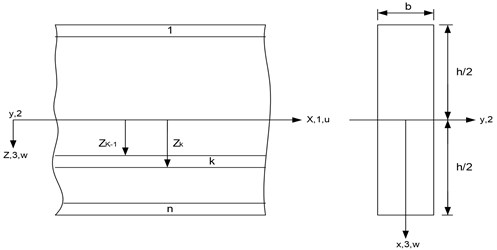Treat the beam as a plane stress problem and employ first-order shear deformation theory. The longitudinal displacement ($U$) and the lateral displacement ($W$) can be written as follows:

(1)
$\left\{\begin{array}{l}U\left(x,z,t\right)=u\left(x,t\right)+z\varphi \left(x,t\right),\\ W\left(x,z,t\right)=w\left(x,t\right),\end{array}\right\$

where $u$ and $w$ are the mid-plane longitudinal and lateral displacements, $\varphi$ is the rotation of the deformed section about the $y$-axis, $z$ is the perpendicular distance from mid-plane to the layer plane, and $t$ is time.

The Strain-Displacement Relations:

(2)
$\left\{\begin{array}{l}{\epsilon }_{1}=\frac{\partial U}{\partial x}=\frac{\partial u}{\partial x}+z\frac{\partial \varphi }{\partial x},\\ {\epsilon }_{5}=\frac{\partial W}{\partial x}+\frac{\partial U}{\partial z}=\frac{\partial w}{\partial x}+\varphi ,\end{array}\right\$

where: ${\epsilon }_{1}$ is the longitudinal strain, and ${\epsilon }_{5}$ is the through-thickness shear strain.

Fig. 2. Composite laminated beam with 3-noded lineal element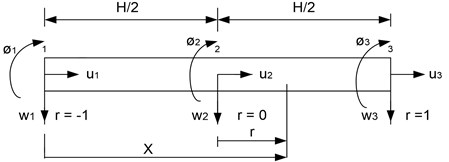By employing 3-noded lineal element as shown in Fig. 2.

The displacements can be expressed in terms of shape function ${N}_{i}$ and nodal displacements:

(3)

The shape functions are: ${N}_{1}=-\frac{r}{2}\left(1-r\right)$, ${N}_{2}=1-{r}^{2}$, ${N}_{3}=\frac{r}{2}\left(1+r\right)$.

From Eqs. (2), (3), the strains can be written as:

(4)
$ϵ=B{a}^{e},$

where:

And ${a}^{e}$ is the vector of nodal displacements ${a}^{e}={\left[\begin{array}{ccc}{u}_{i}& {w}_{i}& {\varnothing }_{i}\end{array}\right]}^{T}$, $i=$ 1, 2, 3.

The stress-strain relation:

(5)
$\sigma =cϵ$,

where $\sigma ={\left[\begin{array}{cc}{\sigma }_{1}& {\sigma }_{5}\end{array}\right]}^{T}$, $ϵ={\left[\begin{array}{cc}{ϵ}_{1}& {ϵ}_{5}\end{array}\right]}^{T}$ and the matrix containing the transformed elastic constants:

$c=\left[\begin{array}{cc}{c}_{11}& 0\\ 0& {c}_{55}\end{array}\right].$

Substitute Eq. (4) in Eq. (5):

(6)
$\sigma =cB{a}^{e}.$

The strain energy:

(7)
${U}_{S}=\frac{1}{2}{a}^{{e}^{T}}b\int {B}^{T}cBdxdz{a}^{e},$
(8)
${U}_{S}=\frac{1}{2}{a}^{{e}^{T}}b{K}^{e}{a}^{e},$

where:

${K}^{e}=\int {B}^{T}cBdxdz$

The kinetic energy:

$T=\frac{1}{2}\int \rho \left[{\left(\stackrel{˙}{u}+z\stackrel{˙}{\varnothing }\right)}^{2}+{\stackrel{˙}{w}}^{2}\right]dv,$

where is density and the dot denotes differentiation with time:

(9)

where:

${M}^{e}=\int \rho {N}^{T}ZNdxdz,$

In the above derivation it is assumed the motion is harmonic and $\omega$ is circular frequency.

In the absence of damping and external nodal load, the total energy is:

The principle of minimum energy requires that:

$\frac{\partial Ԉ}{\partial {a}^{e}}=0.$

The condition yields the equation of motion:

${K}^{e}{a}^{e}-{\omega }^{2}{M}^{e}{a}^{e}=0,$
(10)
$\left[K-{\omega }^{2}M\right]a=0,$

where:

and $n$ is number of elements. To facilitate the solution of Eq. (10), we introduce the following quantities:

where ${K}_{f}$ is the shear correction factor.

The transformed elastic constants are:

In which:

And $\theta$ is the angle of orientation of the ply with respect to the beam axis:

$\left[{I}_{1},{I}_{2},{I}_{3}\right]={\sum }_{k=1}^{n}\underset{{Z}_{k-1}}{\overset{{Z}_{k}}{\int }}\rho \left[1,Z,{Z}^{2}\right]dz.$

Non-dimensional quantities used in the analysis are:

The element stiffness matrix:

${K}^{e}=\int {B}^{T}cBdxdz,$
${K}^{e}=\int \left[\begin{array}{ccc}{A}_{11}\frac{d{N}_{i}}{dx}\frac{d{N}_{j}}{dx}& 0& {B}_{11}\frac{d{N}_{i}}{dx}\frac{d{N}_{j}}{dx}\\ 0& {A}_{55}\frac{d{N}_{i}}{dx}\frac{d{N}_{j}}{dx}& {A}_{55}\frac{d{N}_{i}}{dx}{N}_{j}\\ {B}_{11}\frac{d{N}_{i}}{dx}\frac{d{N}_{j}}{dx}& {A}_{55}{N}_{i}\frac{d{N}_{j}}{dx}& {D}_{11}\frac{d{N}_{i}}{dx}\frac{d{N}_{j}}{dx}+{A}_{55}{N}_{i}{N}_{j}\end{array}\right]dx.$

The mass matrix is 9×9 symmetrical matrix:

${M}^{e}=\int \left[\begin{array}{ccc}{I}_{1}{N}_{i}{N}_{j}& 0& {I}_{2}{N}_{i}{N}_{j}\\ 0& {I}_{1}{N}_{i}{N}_{j}& 0\\ {I}_{2}{N}_{i}{N}_{j}& 0& {I}_{3}{N}_{i}{N}_{j}\end{array}\right]dx.$

#### 3.1. Effect of aspect ratios

Two aspect ratios are given in the numerical results, which are 10 and 50. The first one is for relatively short-thick beams, while the other is for slender beams. The results of the non-dimensional frequencies obtained from the aspect ratio 10 are generally much less than those obtained from the other aspect ratio 50 for same fiber orientation. For example, the fundamental mode of the non-dimensional natural frequencies for a symmetric [30/–30/–30/30] angle-ply hinged _hinged beam with immovable ends is 1.9918 for the aspect ratio 10, and 2.1947 for the aspect ratio 50 as can be seen in Table 1.

This observation can be seen in Fig. 3 to Fig. 5 for symmetric [45/–45/–45/45] angle-ply laminated beams. These figures show the variation of the non-dimensional frequencies with the aspect ratio range from 5 to 40 for the first three modes of vibration for all beams with immovable ends. It is obvious from the figure that the frequency increases rapidly for the range of aspect ratio from 5 to 20, and slows down beyond this range. When the aspect ratio is greater than 20, the beam is slender and consequently shear deformation and rotary inertia have small noticeable effects on the natural frequencies.

Table 2 shows the effect of aspect ratio in non-dimensional frequencies for symmetric [45/–45/–45/45] angle-ply beams. The percentage increase in the non-dimensional frequencies, for the first range of the aspect ratio, increases sharply as the mode order increased for all boundary conditions. For the second range, the percentage increase in frequencies is independent on the mode order. The longitudinal modes of free vibration are also affected by the change of aspect ratio. Generally, the frequencies of longitudinal vibration increase as the aspect ratio increased.

Table 1. Non-dimensional natural frequencies $\left[\overline{\omega }=\omega \sqrt{\rho {L}^{4}/{E}_{1}{h}^{2}}\right]$ [30/–30/–30/30] composite beams with different aspect ratio

 Mode No. Beam type aspect ratio ($L/h=$ 10) CF HH CC HC HF FF 1 0.7465 1.9918 3.4380 2.7113 3.0503 4.3728 2 3.7279 6.4128 7.4386 6.9645 7.9206 9.5329 3 8.4193 11.4744 12.0720 11.7816 12.1545* 14.9573 4 12.1545* 16.5865 16.9085 16.7518 13.1707 20.2046 5 13.4194 21.6353 21.8199 21.7278 18.3742 24.3090* 6 18.5147 24.3090* 24.3090* 24.3090* 23.4730 25.3532 7 23.5463 26.6166 26.7237 26.6709 28.4805 30.3492 8 28.5288 31.5448 31.6114 31.5778 33.4158 35.3042 9 33.4400 36.4344 36.4743 36.4548 36.4635* 40.0877 10 36.4635* 41.2968 41.3227 41.3093 38.2900 44.9060 11 38.3118 46.1413 46.1540 46.1482 43.0983 48.6181* 12 43.1022 48.6181* 48.6181* 48.6181* 47.7785 48.9418 Mode No. Beam type aspect ratio ($L/h=$ 50) CF HH CC HC HF FF 1 0.7837 2.1947 4.8908 3.4027 3.4251 4.9666 2 4.8503 8.6633 13.1481 10.8187 10.9431 13.4813 3 13.3186 19.0823 24.9877 21.9844 22.3328 25.8305 4 25.4001 32.9800 39.8324 36.3924 37.1019 41.4535 5 40.6240 49.8113 57.1574 53.4997 54.6965 59.7934 6 58.4417 69.0255 76.4775 72.7861 60.7725* 80.3045 7 60.7725* 90.1153 97.3759 93.7899 74.5680 102.4978 8 78.3401 112.6436 119.5087 116.1227 96.2177 121.5450* 9 99.8689 121.5450* 121.5450* 121.5450* 119.2209 125.9612 10 122.6521 136.2519 142.5999 139.4697 143.2325 150.3635 11 146.3874 160.6577 166.4325 163.5836 167.9831 175.4481 12 170.8386 185.6456 190.8379 188.2740 182.3175* 201.0230 (*) Modes with predominance of longitudinal vibration

Table 2. The effect of aspect ratio in non-dimensional frequencies for symmetric [45/–45/–45/45] angle-ply beams

 Beamtype Approximate % increase in non-dimensional frequencies Aspect ratios from 5 to 20 Aspect ratios from 20 to 40 1st. mode 2nd. mode 3rd. mode 1st. mode 2st. mode 3rd. mode CF 25 50 100 10 6 9 HH 25 62 100 10 6 9 CC 68 120 150 10 10 15 HC 45 85 125 10 9 14 HF 22 70 220 8 6 10 FF 85 115 145 12 8 12

#### 3.2. Effect of axial movements of the ends

From the results of Table 1, that the values of the non-dimensional frequencies of the transverse modes are not affected by the longitudinal movements of the ends since these modes are generated by lateral movements only (at the yellow shaded). However, the values of the natural frequencies of longitudinal modes are found to be the same for all beams with movable ends since they are generated by longitudinal movements only. Table 3 shows this observation for symmetric [60/–60/–60/60] laminated beams with aspect ratio of 10.

Fig. 3. Effect of aspect ratio on natural frequencies of a symmetric [45/–45/–45/45] cross-play clamped-free beam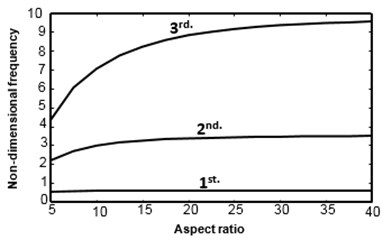Fig. 4. Effect of aspect ratio on natural frequencies of a symmetric [45/–45/–45/45] cross-play clamped-clamped beam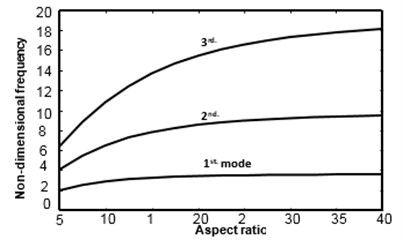Fig. 5. Effect of aspect ratio on natural frequencies of a symmetric [45/–45/–45/45] cross-play free-free beam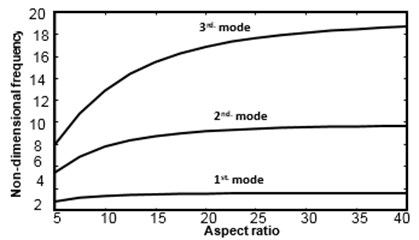Table 1 also shows the fundamental modes of longitudinal vibration for various beams with immovable ends for the symmetric case [30/–30/–30/30] and for two aspect ratios, 10, and 50. It could be noticed that the values of non-dimensional natural frequencies of the longitudinal vibration for the clamped-free and hinged-free beams are equal, and those of the other beams are also the same. This phenomenon occurs since both clamped-free and hinged-free beams with immovable ends are the same when restricted from executing longitudinal motion at the ends. Similarly, the rest of beams with immovable ends have the same longitudinal end conditions.

Table 3. The first two non-dimensional modes of longitudinal free vibration of [60/–60/–60/60] laminated beams with aspect ratio 10

 Beam ends Mode No. Beam type CF HH CC HC HF FF Immovable 1 5.6426 11.2852 11.2852 11.2852 5.6426 11.2852 2 16.9279 22.5705 22.5705 22.5705 16.9279 22.5705 Movable 1 11.2852 11.2852 11.2852 11.2852 11.2852 11.2852 2 22.5705 22.5705 22.5705 22.5705 22.5705 22.5705

#### 3.3. Verification

The natural frequencies results which obtained by this study are closer with Abramovich  results, as show in Table 4, and difference between two results less than 0.6 % for cantilever and clamp-clamp beams.

A third-order shear deformation theory was used by Kant et al.  in the analysis of the free vibration of composite and sandwich simply supported beams. Two comparisons of non-dimensional natural frequencies between the present method (using FSDT) and the results of this reference are presented in Table 5, which presented a comparison for symmetric [0/90/90/0] cross-ply laminated beams respectively, with aspect ratio of ($L/h=$ 5), where the shear effect is significant. The comparison shows a difference of less than 3.3 % associated with the fundamental frequency and less than 4.5 % for higher modes. These differences are due to the employment of different shear theories as stated bellow.

Table 4. Non-dimensional frequencies of [0/90/90/0] composite beams with immovable ends and aspect ratio 10

 Mode No. Cantilever Clamp- clamp Present Ref.  Present Ref.  1 0.8866 0.8819 3.6855 3.7576 2 4.1062 4.0259 7.7244 7.8718 3 8.9536 9.1085 12.381 12.573 4 11.504 12.193 17.192 17.373 5 13.924 14.080 22.119 22.200 6 18.980 18.980 23.007* 23.007

Table 5. Non-dimensional frequencies of [0/90/90/0] composite beams with simple support ends and aspect ratio 5

 Mode No. Present Ref.  1 1.7619 1.820 2 4.2749 4.528 3 6.7214 7.201 4 9.1414 9.814 5 11.5783* – (*) Mode with predominance of longitudinal vibration

It is clear, from the above comparisons, that the differences are very small even for higher modes. This confirms the accuracy of the method of analysis and the computer program.

#### 4. Conclusions

In this paper, free vibration of four layered composite beams has been studied. Both secondary effects of transverse shear deformation and rotary inertia were included in the analysis. A first-order shear deformation theory was applied in the analysis. A finite element model has been formulated to predict the non-dimensional natural frequencies and to study the influence of aspect ratio and movable ends of fibers on the natural frequencies. Different end conditions were studied which are clamped-free, hinged-hinged, clamped-clamped, hinged-clamped, hinged-free, and free-free beams with immovable and movable ends. The main conclusion is the natural frequencies of a laminated beam generally increase with the aspect ratio and all beams with movable ends have equal longitudinal frequencies of vibration, while those of beams with immovable ends are different. Namely, clamped-free and hinged-free beams with immovable ends have equal longitudinal frequencies, and the other beams have also equal longitudinal frequencies.

1. Subramanian P. Dynamic analysis of laminated composite beams using higher order theories and finite elements. Composite Structures, Vol. 73, Issue 3, 2006, p. 342-353. [Publisher]
2. Jafari Talookolaei R.-A., Abedi M., Attar M. In-plane and out-of-plane vibration modes of laminated composite beams with arbitrary lay-ups. Aerospace Science and Technology, Vol. 66, 2017, p. 366-379. [Publisher]
3. Pagani A., Carrera E., Boscolo M., Banerjee J. Refined dynamic stiffness elements applied to free vibration analysis of generally laminated composite beams with arbitrary boundary conditions. Composite Structures, Vol. 110, 2014, p. 305-316. [Publisher]
4. Rueppel M., Rion J., Dransfeld C., Fischer C., Masania K. Damping of carbon fibre and flax fibre angle-ply composite laminates. Composites Science and Technology, Vol. 146, 2017, p. 1-9. [Publisher]
5. Torabi K., Shariati Nia M., Heidari Rarani M. Experimental and theoretical investigation on transverse vibration of delaminated cross-ply composite beams. International Journal of Mechanical Sciences, Vols. 115-116, 2016, p. 1-11. [Publisher]
6. He G., Wang D., Yang X. Analytical solutions for free vibration and buckling of composite beams using a higher order beam theory. Acta Mechanica Solida Sinica, Vol. 29, Issue 3, 2016, p. 300-315. [Publisher]
7. Asl M. E., Niezrecki C., Sherwood J., Avitabile P. Vibration prediction of thin-walled composite I-beams using scaled models. Thin-Walled Structures, Vol. 113, 2017, p. 151-161. [Publisher]
8. Asl M. E., Niezrecki C., Sherwood J., Avitabile P. Similitude analysis of composite I-beams with application to subcomponent testing of wind turbine blades. Experimental and Applied Mechanics, Vol. 4, 2016, p. 115-126. [Search CrossRef]
9. Asl M. E., Niezrecki C., Sherwood J., Avitabile P. Predicting the vibration response in subcomponent testing of wind turbine blades. Special Topics in Structural Dynamics, Vol. 6, 2015, p. 115-123. [Search CrossRef]
10. Vo T. P., Lee J. Flexural-torsional coupled vibration and buckling of thin-walled open section composite beams using shear-deformable beam theory. International Journal of Mechanical Sciences, Vol. 51, Issue 9, 2009, p. 631-641. [Publisher]
11. Algarray A. F. A., Jun H., Mahdi I. E. M. Effects of end conditions of cross-ply laminated composite beams on their dimensionless natural frequencies. Journal of Applied Mechanics and Technical Physics, Vol. 58, Issue 6, 2017, p. 1108-1114. [Publisher]
12. Abramovich H., Livshits A. Free vibrations of non-symmetric cross-ply laminated composite beams. Journal of Sound and Vibration, Vol. 176, Issue 5, 1994, p. 597-612. [Publisher]
13. Marur S. R., Kant T. Transient dynamics of laminated beams: an evaluation with a higher-order refined theory. Composite Structures, Vol. 41, Issue 1, 1998, p. 1-11. [Publisher]